## Articulo

•Citado por SciELO
•Accesos

•Similares en SciELO

## versión On-line ISSN 1390-6542versión impresa ISSN 1390-9363

### Enfoque UTE vol.9 no.4 Quito oct./dic. 2018

#### http://dx.doi.org/10.29019/enfoqueute.v9n4.403

Categoria

A fuzzy sliding mode for Planar 4-Cable Direct Driven Robot

Controlador por modos deslizantes difuso para un robot planar accionado por cuatro cables

1 Escuela Politécnica Nacional, Quito, Ecuador xavier.aguas@epn.edu.ec, andres.cuaycal@epn.edu.ec, israel.paredes@epn.edu.ec, marco.herrera@epn.edu.ec.

Abstract:

Cable Direct Driven Robots (CDDRs) are a special class of parallel robots but they are formed by replacing all the supporting rigid links with cables. Compare with traditional robots, these robots are good candidates for performing a wide range of potential applications. A Planar CDDR model is considered in this paper since no rotational move and no moment resistance are required on the end-effector, all 4 cables convene in a single point and the end-effector is modeled as a point mass. The main goal of this paper is to present a new approach in control by developing a Sliding Mode Controller (SMC) with a Fuzzy-PI as sliding surface using Fuzzy logic toolbox in Matlab/Simulink. The tests performed were Step change reference test and Tracking trajectory test to observe the behavior of the cables during the trajectory and the end-effector movement. Simulation was carried out on Planar 4-Cable CDDR to prove the effectiveness of the proposed control law and the results were compared with a PI Controller and a conventional SMC in terms of integral square error (ISE) index. Only the kinematic model of Planar 4-Cable CDDR is considered in this paper.

Keywords: cable direct driven robot; sliding mode control; Fuzzy controller; PI controller; kinematic.

Resumen:

Palabras clave: robot accionado por cables; control por modos deslizantes; controlador difuso; controlador PI; cinemática.

1. Introduction

2. Methodology

2.1. Model of Planar 4-Cable Direct Driven Robot

In this paper, we use only the reverse kinematic pose solution (Williams Ii & Gallina, 2003). Given the position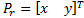is found the cable lengths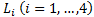. The end-effector position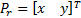is possible to get by geometrical considerations (Euclidean Norm) with each fixed ground link vertex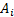(Motor Position). In Figure 1 shows the Planar 4-Cable CDDR scheme. It is important to mention which each cable angle depend of quadrant.

The reverse kinematics pose solution is given in Equation 1,

and the cable angles are given by Equation 2.

Considering the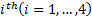cable vector-loop closure equation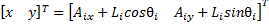is calculated in the velocity kinematics Equations 3 and 4.

Inverting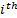cable Jacobian matrix we have Equation 4.

In order to build the inverse velocity solution of Planar 4-Cable CDDR (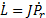) is necessary to eliminate the second row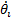as (Williams Ii & Gallina, 2003) to relation the cable length rates and the end-effector velocity as shown in Equation 5.

The alternate forward velocity solution with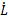as inputs, is calculated through the left pseudoinverse Equation 6,

where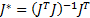is the left pseudoinverse.

2.2. Design of controllers for Planar 4-Cable Cable Direct Driven Robot

This section designs different controllers for Planar 4-Cable (CDDR) based on the kinematic model. The designed controllers are a PI Controller, a conventional SMC and a Fuzzy Logic Controller.

2.2.1. PI Controller

In order to design a PI controller, it is necessary to know the error. The control scheme of this controller is presented in Figure 2.

The tracking error vector is defined in Equation 7,

where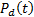is the desired position vector and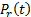is the robot position vector.

This controller has the form given in Equation 8.

Applying this controller to the kinematic model of Planar 4-Cable CDDR is necessary to multiply by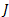, getting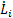as control actions, as in Equation 9,

where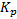and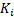are tuning parameters. These parameters have been selected by trial and error until achieving the lowest ISE index.

2.2.2. Sliding Mode Controller

This section shows the design of a SMC with a PI sliding surface (See Figure 3) from the kinematic model of Planar 4-Cable CDDR as in (Villacres, Herrera, Sotomayor, & Camacho, 2017) designs a conventional SMC with a PID sliding surface.

In order to design a conventional SMC, this sliding surface is considered as in Equation 10,

whereis the order of the system andis a positive constant.

This controller has two components: a continuous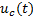and a discontinuous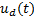, shown in Equation 11.

The system studied is the first order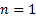for this reason the derivative part of the surface is eliminated. The surface is expressed as in Equation 12.

Now, the surface must be derived for the development of the controller (Equation 13).

By substituting Equation 7 in Equation 13 we have Equation 14.

By replacing Equation 6 in Equation 14,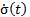it can be rewritten as in Equation 15.

The continuous part of the controller is provided with the condition to keep the output on the sliding surface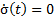and considering the control law as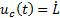(Equation 16).

By completing the SMC control law, the discontinuous part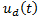is added in Equation 17,

where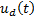is responsible for reaching sliding surface and is composed of a non-linear function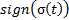which switches about the sliding surface and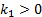is a tuning parameter. These considerations were taken from (Herrera, 2017).

In order to designa positive-definite Lyapunov function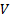is defined in Equation 18.

The derivative of the functionmust be negative-definite (Equation 19).

By replacing equation 15,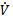is as in Equation 20.

The control law is defined as and substituting we have Equation 21.

To satisfy Equation 19 and replacing Equation 16 in Equation 21,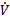should be as in Equation 22,

Therefore, by analyzing (Equation 23):

Finally, to reduce the chattering effect (Camacho & Smith, 2000),can be rewritten as a sigmoid function in Equation 24,

where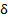is a chattering parameter reduction.

2.2.3. Fuzzy - Sliding Mode Controller

In this section, PI-Fuzzy sliding surface is proposed to a conventional SMC. The selection of this surface is based on the qualitative knowledge about the process to be controlled and was designed using Fuzzy logic toolbox in Matlab/Simulink. For the surface design, there are two inputs and one output. The surface is defined by Equation 25.

The control scheme of Fuzzy-SMC Controller is shown in Figure 4.

The Member Functions (MFs) are namely, NB (Negative Big), NM (Negative Medium), NS (Negative Small), ZE (Zero), PS (Positive Small), PM (Positive Medium), and PB (Positive Big) which are defined as symmetric triangles having 50% overlap. These MFs are shown in Figure 5. For the two input variables (error and integral error), the range for universe of discourse is [-2 2] and for the output variable (action control), it is defined in the range [-0.6 0.6] (Ghosh et al., 2015).

The selected rules for the sliding surface are listed in Table 1. The forty-nine fuzzy rules are based on sliding mode principle (Palm, 1992).

3. Simulation results

The controllers were implemented in Matlab/Simulink 2017a using the kinematic model of Planar 4-Cable CDDR. The tests were run on a computer with an Intel(R)Core(TM) i7-5500U CPU @ 2.40GHz with 8,00 GB RAM, running Windows 10. Figure 6 shows the simulator developed to observe the behavior of the cables during the trajectory. Two tests were performed:

Step Change Reference Test

Tracking Trajectory Test

The simulation had a duration of 110 seconds with a sampling time of 0.1 for each test and uses ODE45 (Solve non-stiff differential equations). The physical parameters of Planar 4-Cable CDDR are presented in Table 2.

Finally, the values ofandfor PI Controller and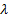,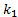and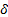for SMC have been selected by trial and error until achieving the lowest ISE index. In Table 3 are shown these values.

We used Equations 26 and 27 to compare the controllers based on ISE index.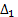corresponds to the comparison between conventional SMC and Fuzzy-SMC Controller and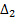corresponds to the comparison between PI Controller and the control law proposed,

where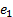,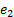and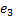represent error values for Fuzzy-SMC Controller, PI Controller and conventional SMC respectively.

3.1. Step change reference test

In this test, a step change reference is made from the reference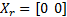to the point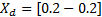. In Figure 6 illustrates the end-effector path.

Figure 7 presents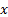and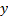end-effector positions during the change reference to the position. The percentage overshoot of Fuzzy-SMC Controller is less than conventional SMC and the setting time is lower than PI Controller.

In Table 4 shows ISE index comparison between three controllers for step change reference test wherein the performance of Fuzzy-SMC Controller is the best according to ISE index.

3.2. Tracking trajectory test: square

In this test, the selected trajectory is a square whose side length is 0,4[m]. In Figure 8 illustrates the efficiency of three designed controllers for this desired trajectory.

In Figure 9 presents an image zoom at beginning and at the corner of the square trajectory, which shows Fuzzy-SMC Controller has lower overshoot than conventional SMC.

In Table 5 shows the ISE comparison between the three controllers for tracking trajectory test.

In all tests, the proposed control law presents the lowest ISE index compared to the two controllers, the first is a PI controller and the other is a conventional SMC controller. In the two tests performed, the movement of the end effector is significantly improved because the fuzzy controller applied to the sliding surface softens the control actions, which are abrupt of the SMC controller.

4. Conclusion

In this paper, PI, SMC and Fuzzy-SMC controllers were designed for end-effector position control of Planar 4-Cable CDDR based on the kinematic model. These controllers were able to perform trajectory tracking and step change reference wherein the results indicate which SMC with Fuzzy-PI as sliding surface with forty-nine rules presents lower setting time than PI controller and lower oscillation than the conventional SMC. The performance of the controller was evaluated in terms of integral square error (ISE) index and these results demonstrate the effectiveness of proposed controller showing an acceptable accuracy.

References

Ataei, M., & Shafiei, S. E. (2008). Sliding mode PID-controller design for robot manipulators by using fuzzy tuning approach. En Proceedings of the 27th Chinese control conference (pp. 16-18). Citeseer. [ Links ]

Babaghasabha, R., Khosravi, M. A., & Taghirad, H. D. (2014). Adaptive Control of KNTU Planar Cable-Driven Parallel Robot with Uncertainties in Dynamic and Kinematic Parameters. Recuperado 26 de octubre de 2018, de /paper/Adaptive-Control-of-KNTU-Planar-Cable-Driven-Robot-Babaghasabha-Khosravi/3cf12282c9a37211f8f7adbc5e667a1436317626 [ Links ]

Camacho, O., & Smith, C. A. (2000). Sliding mode control: an approach to regulate nonlinear chemical processes. ISA Transactions, 39(2), 205-218. https://doi.org/10.1016/S0019-0578(99)00043-9Links ]

Gallina, P., Rossi, A., & Williams II, R. L. (2001). Planar cable-direct-driven robots, part ii: Dynamics and control. En ASME. DECT2001 ASME Design Engineering Technical Conference. Pittsburgh: ASME Publisher (Vol. 2, pp. 1241-1247). [ Links ]

Ghosh, A., Sen, S., & Dey, C. (2015). Design and real-time implementation of a fuzzy PI controller on a servo speed control application. En Signal Processing and Integrated Networks (SPIN), 2015 2nd International Conference on (pp. 384-387). IEEE. [ Links ]

Herrera, M. (2017). A Blended Sliding Mode Control with Linear Quadratic Integral Control based on Reduced Order Model for a VTOL System. Recuperado de http://repositorio.educacionsuperior.gob.ec/handle/28000/4641Links ]

Jin, X., Jun, D. I., Pott, A., Park, S., Park, J.-O., & Ko, S. Y. (2013). Four-cable-driven parallel robot. 879-883. [ Links ]

Khosravi, M. A., & Taghirad, H. D. (2014). Robust PID control of fully-constrained cable driven parallel robots. Mechatronics, 24(2), 87-97. [ Links ]

Nabi, A. (2013). Design of fuzzy logic PD controller for a position control system. International Journal of Engineering and Management Research, 3(2). [ Links ]

Oh, S.-R., & Agrawal, S. K. (2003). Cable-suspended planar parallel robots with redundant cables: Controllers with positive cable tensions. En IEEE International Conference on Robotics and Automation (Vol. 3, pp. 3023-3028). IEEE; 1999. [ Links ]

Palm, R. (1992). Sliding mode fuzzy control. En Fuzzy Systems, 1992., IEEE International Conference on (pp. 519-526). IEEE. [ Links ]

Villacres, J., Herrera, M., Sotomayor, N., & Camacho, O. (2017). A fuzzy sliding mode controller from a reduced order model: A mobile robot experimental application. En Control, Decision and Information Technologies (CoDIT), 2017 4th International Conference on (pp. 0674-0678). IEEE. [ Links ]

Williams Ii, R. L., & Gallina, P. (2003). Translational planar cable-direct-driven robots. Journal of Intelligent and Robotic systems, 37(1), 69-96. [ Links ]

Williams, R. L., Gallina, P., & Rossi, A. (2001). Planar cable-direct-driven robots, part i: Kinematics and statics. En Proceedings of the 2001 ASME Design Technical Conference, 27th Design Automation Conference (pp. 178-186). [ Links ]

Zanotto, D. (2011). Analysis and development of cable-driven robotic devices. [ Links ]

Received: May 15, 2018; Accepted: November 05, 2018This is an open-access article distributed under the terms of the Creative Commons Attribution License